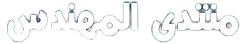# Can anyone help me with elgamal algorithm plz

## p=23 g=11 a=6 y=mod(g^a,p) k=3 m=10 % represents message i want to encrypt before sending it. y1=mod(g^k,p) %first block of encryption process y2=mod(m*y^k,p) %second block of encryption process

result=mod(y2*(y1^a)^-1,p) % result from this step must be the encrypted message which here equal to 10 but i received wrong answer, can any one please help me to fix it

انتظر ردكم يا شباب على الكود واتمنى اني الاقي الحل عندكم لاني بصراحه تعبت وانا احاول احل المشكله لكن للاسف ما عرفت احلها وانا لازم اسوي البرنامج

أخى العزيز فى الكود الذى ذكرته انت استعملت

``````y=mod(g^a,p)
``````

وهذا غير صحيح فى ألجوريثم الجمل
اولا لانه يجب ان يكون على هذه الصورة

``````y = g^a mod p
``````

وفى هذه الحالة mod يعنى Primitive root modulo وعند استعمالك لها بالطريقة التى ذكرتها فانك تقوم بحساب المتبقى من القسمة
فعلى سبيل المثال عند تنفيذ الكود الاتى

``````p=11
g=4
a=2
y = g^a mod p
``````

فيكون الناتج y=3

ويمكنك حساب Primitive root modulo من خلال المثال الموجود على الرابط الاتى
http://www.math.mtu.edu/mathlab/COURSES/holt/dnt/quadratic4.html
او ويكيبيديا
http://en.wikipedia.org/wiki/Primitive_root_modulo_n

السلام عليكم

الله يعطيك العافيه اخي الكريم على ردك على موضوعي والان سؤالي هو كيف يمكن كتابة الصيغة التي قلتها بلغة الماتلاب؟؟؟!!!

انا حاولت كتابة الصيغة التاليه وهي:

y = g^a mod p

وكانت النتيجه هي ظهور رسالة خطا هي:

y=g^a mod p
??? y=g^a mod p
|
Error: Missing operator, comma, or semicolon.

اتمنى يا اخي الكريم انك تكون فهمت سؤالي وتخبرني بكيفية كتابة الصيغة السابقه بالماتلاب ولك جزيل الشكر

أخى العزيز لازم تفهم الاول كيفية حساب Primitive root modulo يدويا علشان تقدر تطبقهم فى الماتلاب وفى هذا الموقع مجموعة دوال ممكن تفيدك
http://www.cs.kuleuven.be/~dirkn/code/

السلام عليكم

الله يجزاك خير ويعطيك الف مليون عافيه ويوفقك دنيا واخره

والله يا الحبيب انا ماني خبير ولا محترف في الماتلاب بس احاول استفيد من اصحاب الخبره الموجودين هنا مثلك وشرواك بس ياليت يا غالي لو تقدر توضح لي بمثال وانا ان شاء الله احاول اطبقه على برنامجي ولك جزيل الشكر

معليش انا عارف اني جالس اغلبك معي بس اتمنى انك تتحملني

الله يعطيك العافيه يا غالي ومعليش اني جالس اتعبك واغلبك معي بس اتمنى انك تصبر معي وتتحملني شوي

انا ماني خبير ولا محترف بالمتلاب بس احاول استفيد من الصحاب الخبره مثلك وشرواك

المهم يا ليت يا غالي توضح لي كيفية كتابة الكود بمثال بسيط وانا لك من الشاكرين

جزاك الله خيرا اخى العزيز على كلامك الطيب لكن فى الحقيقة انا حاسس انك مش فاهم Primitive root modulo او مقراءتش الموضوع
المهم انك لازم تفهم Primitive root modulo كويس جدا انا بصراحة معنديش وقت اقراءه علشان اعرف طريقة عمله لكن انت ممكن تستعمل الدالة الموجود فى الموقع اللى ذكرة مباشرة لحساب الجذر الاولى او على الاقل ممكن تعرف طريقة عمل الدالة وتحاول تدمجها فى برنامجك

السلام عليكم

انا حملت الداله من الموقع يا غالي وعند تطبيق البرنامج تظهر رسالة الخطأ التاليه:::

p=23
g=11
a=6
z=powmod(g,a,p)
p =
23

g =
11

a =
6
??? Error: File: C:\MATLAB6p1\work\powmod.m Line: 23 Column: 39
Expected a variable, function, or constant, found “)”.

ممكن تقلي يا غالي ايش معنى رسالة الخطأ السابقه وكيف ممكن احل المشكله هذه ولك جزيل الشكر

ابعتلى البرنامج اللى بتنفذه وكل الدوال اللى معتمده عليه علشان اقدر انفذه عندى واشوف الخطأ
وياريت تستخدم وسوم الكود الموجودة فى المحرر